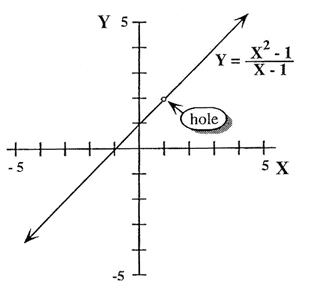# How to determine a hole in a graph?

I'm aware that in order to find the hole in a graph, you need to factor both the numerator and denominator, and look for terms that cancel out.

However, is it merely just looking for a term that cancels out, or is it more specifically a term that cancels out and makes the numerator equal to zero?

Can you clarify what you mean by a "hole" in a graph? If you mean points where the graph is not defined, then there are various scenarios where there can be non-continuity in the domain.

Can you clarify what you mean by a "hole" in a graph? If you mean points where the graph is not defined, then there are various scenarios where there can be non-continuity in the domain.

Something like thisEdit: Actually, this picture might have just answered my question.

So we have the equation: $\frac{x^2-1} {x-1}$

which reduces to: $\frac{(x+1)(x-1)} {(x-1)}$

The $(x-1)$ terms will both cancel out, but that still leaves the numerator as a non-zero value. Thus, maybe it's merely just a term that cancels out that causes a hole. In this case, the term is $(x-1) = (x = 1)$ which corresponds with the hole in the graph at $x = 1$

Last edited:
Stephen Tashi
It's hard to express your idea with mathematical precision. To put your question in a sophisticated way: When does a function that is the ratio of polynomial factors have a "removable singularity"? You want to know when an otherwise solid graph of a function has a hole at a (finite) point (x,y).

One can define funtions in complicated ways using if...then rules as well as algebraic expressions. Suppose we only consider a function defined by the ratio of polynomial factors. (i.e. its is a single fraction , not a sum of several fractions).

Functions like $f(x) =\frac{(x^2 + 1) (2x + 3)} { (x^2 + 1)}$ don't have a hole in their graph because (in the real number system) there is no value of $x$ that would make the denominator zero. So the fact that the numerator and denominator have a common factor does not always imply the function has a hole in its graph. Looking for terms that cancel out, doesn't automatically locate a hole in the graph.

By contrast, the graph of the function $g(x) = \frac{(x^2 -1 )(2x + 3)}{(x^2 - 1) }$ has a holes when $x = 1$ and $x = -1$.

The graph of the function $h(x) = \frac{ 6 + (x^2 )}{(x^2-1) }$ doesn't exist at the values $x = 1$ and $x = -1$ because those values make the denominator zero. Since $x^2 -1$ is not a common factor, the fraction cannot be reduced. The graph does not have a hole at any finite point (x,y). Values of $x$ that make the denominator zero cause the graph not to exist, even if there are no common factors in the fraction.

It's hard to express your idea with mathematical precision. To put your question in a sophisticated way: When does a function that is the ratio of polynomial factors have a "removable singularity"? You want to know when an otherwise solid graph of a function has a hole at a (finite) point (x,y).

One can define funtions in complicated ways using if...then rules as well as algebraic expressions. Suppose we only consider a function defined by the ratio of polynomial factors. (i.e. its is a single fraction , not a sum of several fractions).

Functions like $f(x) =\frac{(x^2 + 1) (2x + 3)} { (x^2 + 1)}$ don't have a hole in their graph because (in the real number system) there is no value of $x$ that would make the denominator zero. So the fact that the numerator and denominator have a common factor does not always imply the function has a hole in its graph. Looking for terms that cancel out, doesn't automatically locate a hole in the graph.

By contrast, the graph of the function $g(x) = \frac{(x^2 -1 )(2x + 3)}{(x^2 - 1) }$ has a holes when $x = 1$ and $x = -1$.

The graph of the function $h(x) = \frac{ 6 + (x^2 )}{(x^2-1) }$ doesn't exist at the values $x = 1$ and $x = -1$ because those values make the denominator zero. Since $x^2 -1$ is not a common factor, the fraction cannot be reduced. The graph does not have a hole at any finite point (x,y). Values of $x$ that make the denominator zero cause the graph not to exist, even if there are no common factors in the fraction.

Thank you, this helped!

Adding to what Stephen said, explicit non-polynomial functions containing terms of the type ##u^{-|R|}## (where ##u## is an expression containing ##x##) have "holes" in their graphs if ##u=0## for any real x value . The simplest function of this type is ##y=x^{-1}## (u=x here) which has an asymptote at x=0. Similarly, by letting u=cos x and R=1 , the function will have multiple "holes" arranged in a recurring fashion wherever cos x = 0 (this will be the natural domain of sec x). I also must add that the concept is not just limited to fractions containing x terms in the denominator, but also logarithmic functions, where ##log_a u## is not defined for any x value where u=0.